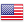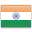Grade "A+" Accredited by NAAC with a CGPA of 3.46
Grade "A+" Accredited by NAAC with a CGPA of 3.46

# Basic Econometrics Using Software

Course ID
MS 407
Level
Program
BMS
Semester
Fourth
Credits
4.0
Paper Type
Skill Enhancement
Method
Lecture & Practical

## Unique Paper Code: Update Awaited

This course provides basic econometric concepts, understanding through software. It covers estimation and diagnostic testing of simple and multiple regression models.

## Learning Outcomes:

At the end of the course, students should be able to:

• Understanding of basic econometrics and its assumptions and impact of violations of classical assumptions
• Interpretation of regression model
• Interpretation of functional forms of regression model.

## Course Contents

Unit I
Unit II
Unit III
Unit IV

Unit I (3 Weeks)

Introduction to Econometrics and an overview of its applications; Simple Regression with Classical Assumptions; Least Square Estimation And BLUE, Properties of estimators, Multiple Regression Model and Hypothesis Testing Related to Parameters – Simple and Joint.

References:

Gujarati, N. Damodar. Basic Econometrics. New Delhi: McGraw Hill. [ Chapter 1-9]

Gujarati, N. Damodar. Econometrics by Examples. New Delhi: McGraw Hill. [Chapter1-3]

Unit II (3 Weeks)

Assumptions Violations; understanding of assumptions, what is the consequences if violated, their identification, how to take care.
Zero Mean of error
• Homoscedasticity
• Autocorrelation
• Uncorrelatedness of regressor and disturbance
• Normality
• Non-Stochastic Regressor

References:

Gujarati, N. Damodar. Basic Econometrics. New Delhi: McGraw Hill. [Chapter 10-13]

Gujarati, N. Damodar. Econometrics by Examples. New Delhi: McGraw Hill. [Chapter 4-7]

Unit III (3 Weeks)

Outliers & Influential Points; understanding, deduction and how to take care. goodness of fit.

References:

Gujarati, N. Damodar. Basic Econometrics. New Delhi: McGraw Hill. [Chapter 3 and 4]

Unit IV (3 Weeks)

How to report results of the Regression?

How to decide which Regression Model provides a better fit?

Interpretation of functional forms of regression model.

Scaling effect in Regression …

Point and Interval Estimation of parameters of the Regression Model

References:

Gujarati, N. Damodar. Basic Econometrics. New Delhi: McGraw Hill. [Chapter 5]

#### Text Books

Christopher Dougherty (2007). Introductory Econometrics (3rd ed.). Oxford University Press.
Gujarati, Damodar Basic Econometrics (4th ed.). New Delhi: McGraw Hill.

Pindyck, Robert S. and Daniel L. Rubinfeld (1997) Econometric Models and Economic Forecasts. (3rd ed.). Singapore: McGraw Hill.
Ramanathan, Ramu (2002). Introductory Econometrics with Applications (5th ed.). Thomson South Western

#### Teaching Learning Process

This course will be taught using a mix of the following tools:
1. Relevant Case studies
2. Hands on practice on stata and R
3. Relevant and important articles from academic linked journals in the domain of Management such as Harvard Business Review,

#### Assessment Methods

Practical + Internal assessment – 50 marks
Written exam – 50 marks

#### Keywords

Prowess, R- software, Stata, Multiple Regression and Classical Assumptions

Disclaimer: Details on this page are subject to change as per University of Delhi guidelines. For latest update in this regard please refer to the University of Delhi website here.Englishहिन्दी